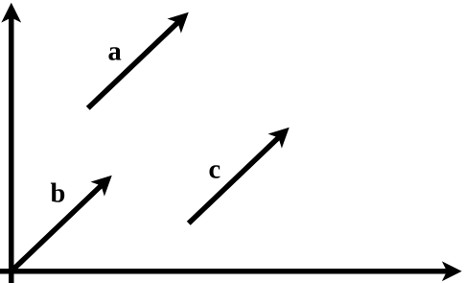# A Short Intuitive Explanation of Vectors

## What is a vector?

I know you might be already thinking- “a vector is something which has magnitude and direction”. But that’s old school definition. I also used to think that way, but after I left the academic world, I had to dig deeper. The more I explored, the deeper the concept became.

This post is going to be some thoughts on this concept. If you have never thought beyond the typical old school definition, I hope this post might bring some new perspective.

### The direction in the abstract sense

The very first time I got dissatisfied with the old school definition was in a signal processing class. A bunch of numbers in an array form were shown, then someone claimed it as a vector. I was thinking like, ok so where does the vector point to? where is the direction in these groups of numbers? does it point towards north/south or any other direction?. These questions made me prob further.

Only later in my career, I came to know that vectors can have multiple definitions or perspectives depending upon the domain. We normally get introduced to the concept from the physics perspective (e.g.something that moves north, an apple falling to the ground, or pushing something in an arbitrary direction). The famous YouTube channel 3blue1brown, from Grant Sanderson, has an extremely good video on the topic. He mainly groups the vector explanation into the computer scientists, physicists, and mathematicians’ perspective.

Suppose my height is 174cms and weight is 80 kg. From a computer scientist’s perspective, these two quantities can form a vector. This very often represented in the form of a column vector as $[174 \hspace{2mm} 80]^T$.

### Some nitty-gritty details

The reason for using $T$ here is to denote transpose operation. Writing it horizontal saves space, but that would make it a row vector. We often want the vectors as column vectors so that it can be easily processed using the rules of linear algebra. Such nitty-gritty details never appear in any textbook but are so important when it comes to really understand a topic.

If I were highly overweight with my current height, I could say that I belong to a category of obese people. In other words, I could say this vector represent/point toward people with an average height but obese. The key insight here is that the direction here is an abstract one and not a physical one like north/south/west/east. This is when it clicked to me why a bunch or numbers called vectors.

The beauty of mathematics comes with such abstraction and generalization. Just like a number ‘3’ can represent three sheep/apples/asteroids/anything, when it comes to vectors, the direction could be abstract. In some cases, we might be lucky to relate an abstract mathematics thing to physical reality, and in most cases, we cannot. For example, I have shown this example with only two elements. It could be possible to have three/four/infinite elements in a vector.

### Visualizing vectors

Coming to visual representation of vectors, we can represent 2D vectors in a plane and somewhat 3D vectors in a space also. But anything above that is beyond our physical reality. Graphical representation of 2D vectors often draws vectors starting from the origin as shown below.

What if I tell you that here all the vectors a, b, and c are the same, do you agree?. All have the same magnitude and direction. It is just that we used to draw it from the origin like vector b shown here. A more precise description of the vector could be a push or force in a certain abstract direction with a specific magnitude.

We could have placed the coordinates rotated any $\theta$ degrees and draw the vector withrespect to that. The essense here is that the vector space in which all vectors exist could come in any form, dimension, abstract directions, and components which represents various qualities or parameters.

Just for an example, any polynomial can be thought of as a vector (e.g. $3x^3+2x^2+5$). Here the coefficients (3, 2, and 5) are the components and the power terms ($x^3$, $x^2$, and $x^0$) represent each dimension/component.

### What is a unit vector?

Another point of confusion that might arise is the magnitude of the unit vector. A unit vector is often defined as one with a magnitude equal to one/unity. As shown in our example, what if the vector components represent different parameters like height (in meters) and weight (in kilograms) ?. Since the magnitude has to be 1, it should satisfy the relation $height^2+weight^2 =1$. Suppose if the height is already 1 meter, the only way to make a unit vector out of it would be to make weight component zero. This is why in cartesian coordinate the unit vectors have all elements zero except the component ($i$, $j$, or $k$) it represents as shown here. $\hat{i}=[1\hspace{2mm}0\hspace{2mm}0]^T$, $\hat{j}=[0\hspace{2mm}1\hspace{2mm}0]^T$, and $\hat{k}=[0\hspace{2mm}0\hspace{2mm}1]^T$.

## Conclusion

The story of vectors never stops. I only tried to address some parts of it which might confuse someone getting introduced to the concept for the first time. The idea of vectors has lot of connections with various subjects in science and engineering. There are a lot of moving parts of it that need careful understanding. I hope this post sheds some new light on your current understanding of vectors. We will see more detailed and related topics in later posts.